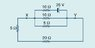# Current & Voltage Homework: Find Current & PD @ X,Y

• TyErd

## Homework Statement

For the circuit shown

Find:
1. The current in the 20ohm resistor
2. The potential difference between points X and Y

V=IR

## The Attempt at a Solution

the resistors in the parallel component were assigned a voltage of 25 and thus found the current to be a total of 10A. When exiting the parallel component it must be 10A thus the 20ohm resistor has a 10A current passing through it.

#### Attachments

•circuit2.JPG
5.9 KB · Views: 390

but can you do it will all three resistors, even though there's a power supply?

...?

Sorry I read the original problem wrong. Treat the parallel section of the circuit as its own serperate circuit. Collapse the three resistors into a single resistance. Then try proceding from there.

by the parallel section do you mean just 10 and the 5 or 10, 5 and the other 10 with the power supply?

even then I'm not sure how to break the circuit up

So, you have the parallel section of the circuit. Treat that as it's own individual circuit. Add the three resitors, then you plug that back into the circuit. So, you end up with the battery, the Req and the 20 and 5 ohm resitors in series. Then find the current and you can find the potential drop.

okay so i did that, and got a current of 0.9A, which is incorrect

?